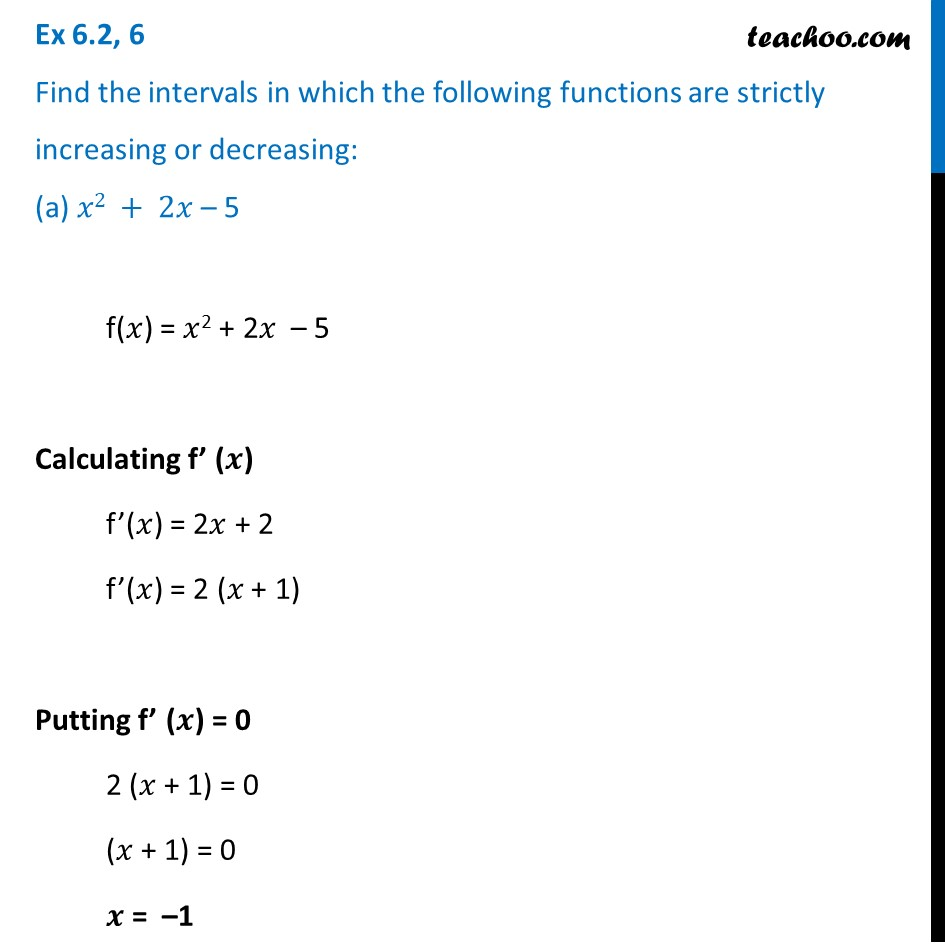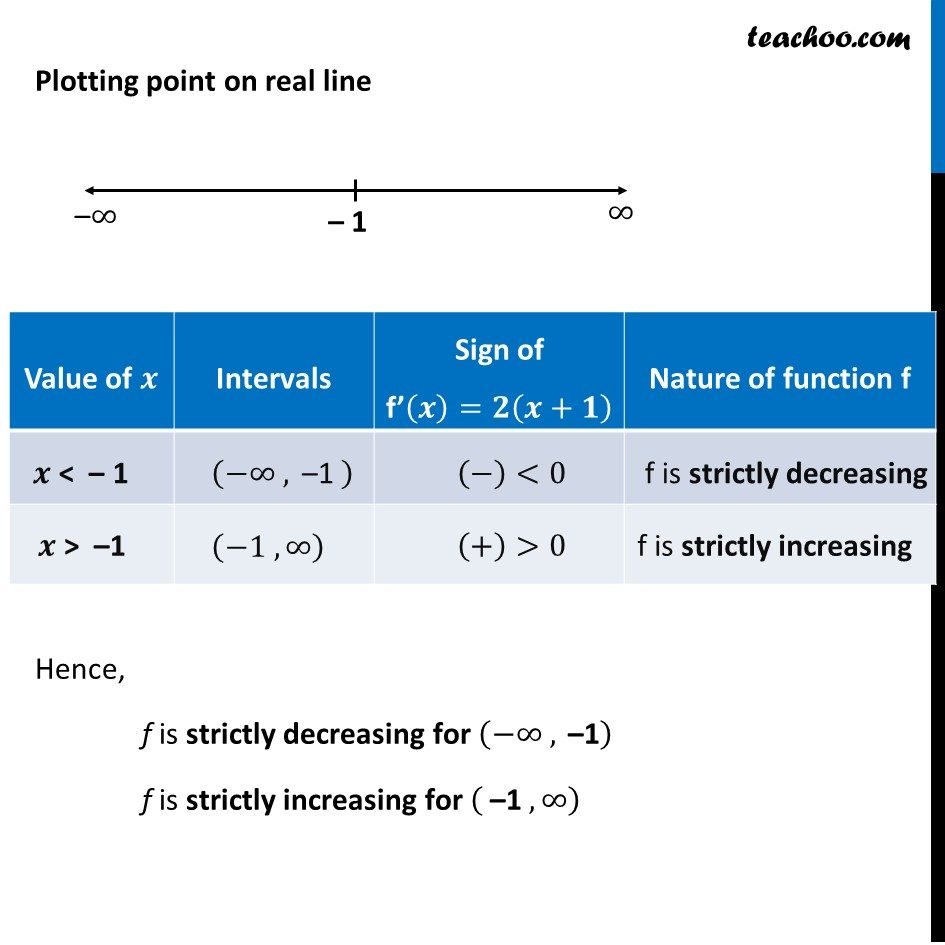Find intervals of increasing/decreasing

Chapter 6 Class 12 Application of Derivatives
Concept wiseIntroducing your new favourite teacher - Teachoo Black, at only ₹83 per month

### Transcript

Ex 6.2, 6 Find the intervals in which the following functions are strictly increasing or decreasing: (a) 𝑥2 + 2𝑥 – 5 f(𝑥) = 𝑥2 + 2𝑥 – 5 Calculating f’ (𝒙) f’(𝑥) = 2𝑥 + 2 f’(𝑥) = 2 (𝑥 + 1) Putting f’ (𝒙) = 0 2 (𝑥 + 1) = 0 (𝑥 + 1) = 0 𝒙 = –1 Plotting point on real line Hence, f is strictly decreasing for (−∞ ," –1" ) f is strictly increasing for ( "–1" ,∞)